Math resources Geometry 2D Shapes

Irregular Polygon

# Irregular polygon

Here you will learn about irregular polygons, including what they are and how to classify them.

Students will first learn about irregular polygons as part of geometry in 2nd grade and 3rd grade.

## What is an irregular polygon?

An irregular polygon is a closed, straight-sided figure that has unequal sides or angle measurements. If a polygon has all equal sides and interior angles, it is a regular type of polygon.

For example,

Regular pentagon: Irregular pentagon: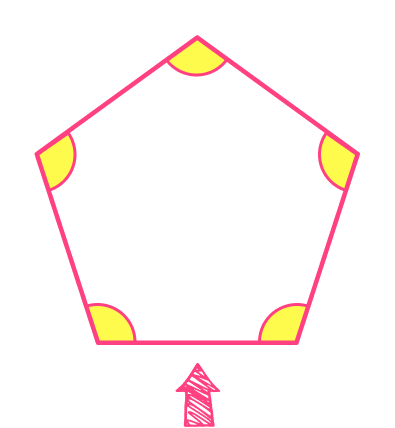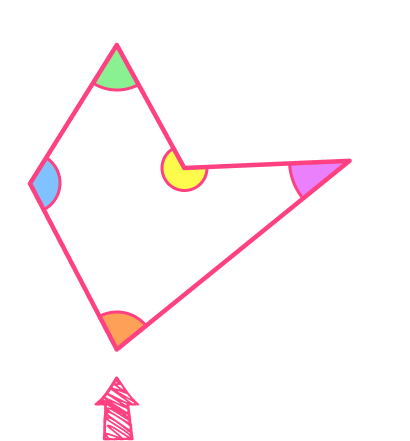All the sides are the same length. All the sides are NOT the same length.

All the angles are the same size. All the angles are NOT the same size.

The table below gives examples of irregular polygons,

PolygonNumber of
sides/vertices
Image

Scalene triangle

\textbf{3}

\textbf{4}

Irregular pentagon

\textbf{5}

Irregular hexagon

\textbf{6}

Irregular octagon

\textbf{8}

### What are irregular polygons?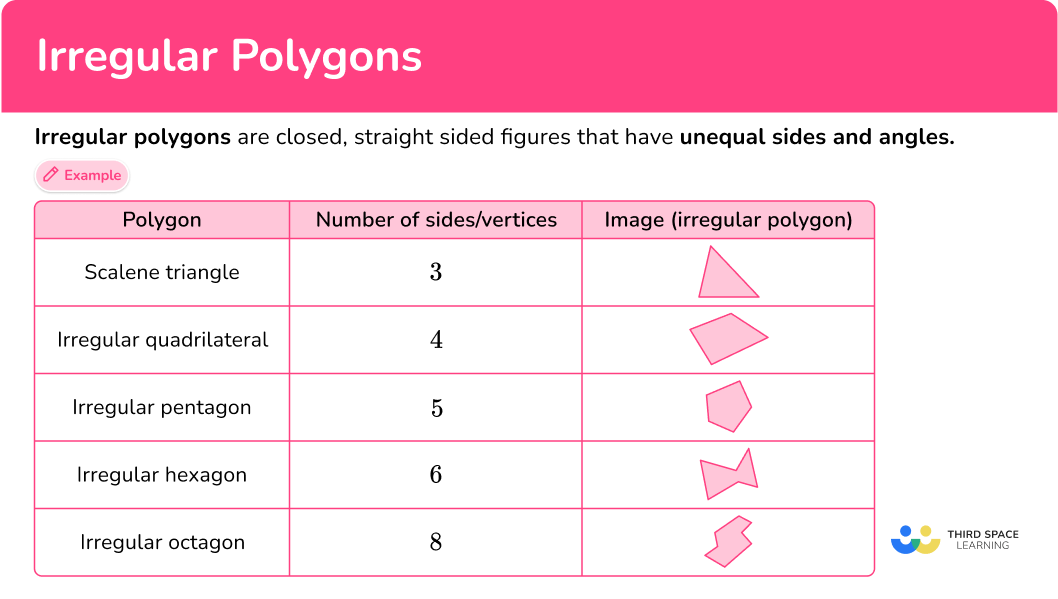## Common Core State Standards

How does this relate to 2nd grade math and 3rd grade math?

• Grade 2 – Geometry (2.G.A.1)
Recognize and draw shapes having specified attributes, such as a given number of angles or a given number of equal faces. Identify triangles, quadrilaterals, pentagons, hexagons, and cubes.

• Grade 3 – Geometry (3.G.A.1)
Understand that shapes in different categories (for example, rhombuses, rectangles, and others) may share attributes (example, having four sides), and that the shared attributes can define a larger category (example, quadrilaterals). Recognize rhombuses, rectangles, and squares as examples of quadrilaterals, and draw examples of quadrilaterals that do not belong to any of these subcategories.

## How to classify an irregular polygon

In order to classify an irregular polygon:

1. Check to see that the polygon has different size angles or sides.
2. Count the number of sides of the polygon.
3. Name the polygon based on the number of sides.

## Irregular polygon examples

### Example 1: classify the shape – by sides and regular or irregular

Name the shape.

1. Check to see that the polygon has different size angles or sides.

There are sides and angles that are not congruent (equal), so the polygon is irregular.

2Count the number of sides of the polygon.

There are 4 sides.

3Name the polygon based on the number of sides.

The polygon is an irregular quadrilateral.

### Example 2: classify the shape – by sides and regular or irregular

Name the shape.

There are sides and angles that are not congruent, so the polygon is irregular.

There are 3 sides.

The polygon is an irregular triangle. More specifically, it is also a right scalene triangle.

### Example 3: classify the shape – by sides and regular or irregular

Name the shape.

There are sides and angles that are not congruent, so the polygon is irregular.

Notice that some angles and sides are congruent, but since not all angles and sides are, the figure is irregular.

There are 8 sides.

The polygon is an irregular octagon.

### Example 4: classify the shape – by sides and regular or irregular

Name the shape.

There are sides and angles that are not congruent, so the polygon is irregular.

Notice that 2 angles are congruent, but since not all angles are, the figure is irregular.

There are 5 sides.

The polygon is an irregular pentagon.

### Example 5: classify the shape – by sides and regular or irregular

Name the shape.

There are sides and angles that are not congruent, so the polygon is irregular.

There are 6 sides.

The polygon is an irregular hexagon.

### Example 6: classify the shape – by sides and regular or irregular

Name the shape.

There are sides and angles that are not congruent, so the polygon is irregular.

There are 6 sides.

The polygon is an irregular hexagon.

### Teaching tips for irregular polygons

• Worksheets can be a good way for students to practice identifying irregular polygons, but it is also important for students to work with shapes in real life. One way to do this is to give students straws that are of different lengths and clay. They can use the straws as line segments and connect them with clay to create irregular polygons.

Once students have created an irregular polygon, they can compare and contrast their polygon with what other students have made, or if they are working on their own, they can draw a picture of it and then create another polygon with the same number of sides, but a different overall shape.

• Highlight irregular polygons that are common in everyday life (at home or at school) and encourage students to notice them as well. Even if they don’t know how to classify a polygon, just naming a shape that is an irregular polygon helps form meaning around this topic.

### Easy mistakes to make

• Thinking that irregular polygons can’t have any equal sides or angles
An irregular polygon can have some equal sides and angles.
For example,
This irregular hexagon does have some equal sides and angles, but they are not ALL equal, so it is irregular. A rhombus is a quadrilateral with four equal sides, however, not all of its angles are equal, therefore a rhombus is not a regular quadrilateral – that is a square.

• Thinking only regular polygons are polygons
Many children’s books, classroom posters, and other commonly used objects show only regular polygons. This makes it easy for students to think that a shape has to be in these exact forms.
For example,
Thinking a 6 sided closed figure is not a hexagon, because it does not look like a regular hexagon.
Seeing and talking about irregular polygons helps correct this misconception.

There are many irregular quadrilaterals and it is common to confuse the properties, especially for a rhombus, parallelogram, or trapezoid. When asked to specify the classification of an irregular quadrilateral, always recall the qualities of each type.

### Practice irregular polygon questions

1. Which of the following is an irregular polygon?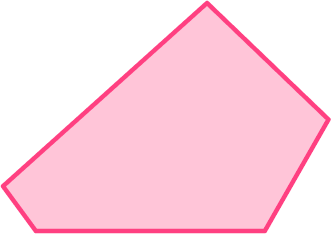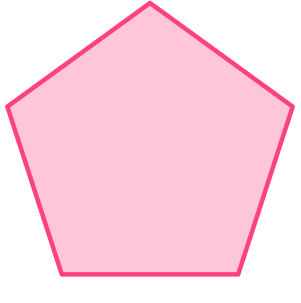Irregular polygons are closed, straight sided figures that have unequal sides and angles.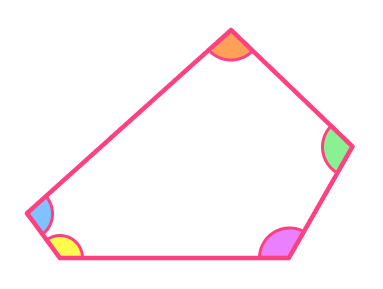The figure is closed, made up of straight sides and has unequal sides and angles.

2. Which of the following is an irregular polygon?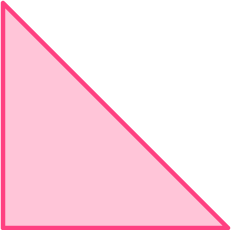Irregular polygons are closed, straight sided figures that have unequal sides and angles.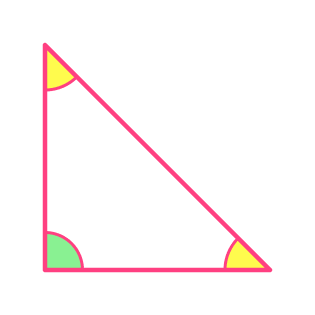The figure is closed, straight sided and has unequal sides and angles.

Even though it has some equal sides and angles, because ALL the sides and angles are not equal, it is irregular.

3. Which of the following is an irregular hexagon?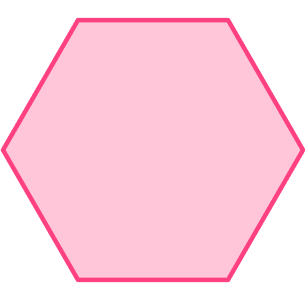An irregular hexagon is a 6 sided closed figure that has unequal sides and angles.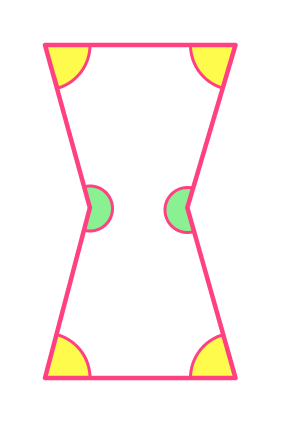The figure is closed and has unequal sides and angles.

Even though it has some equal sides and angles, because ALL the sides and angles are not equal, it is irregular.

4. Which of the following is an irregular quadrilateral?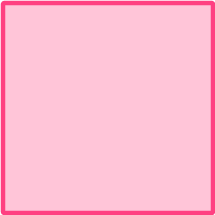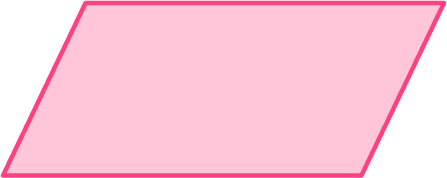An irregular quadrilateral is a 4 sided closed figure that has unequal sides or angles.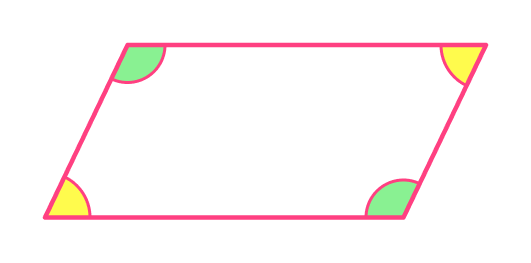The figure is closed and has unequal sides or angles.

Even though it has some equal sides and angles, because ALL the sides and angles are not equal, it is irregular.

5. What is the name of the figure?irregular hexagonoctagonirregular pentagon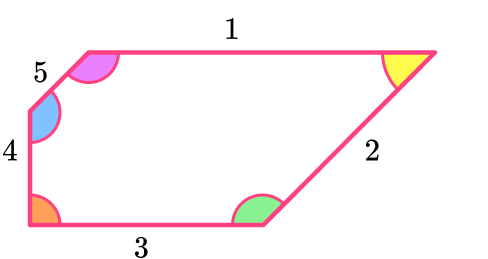An irregular pentagon is a 5 sided closed figure that has unequal sides and angles.

6. What is the name of the figure?irregular octagonirregular hexagonpentagonirregular triangle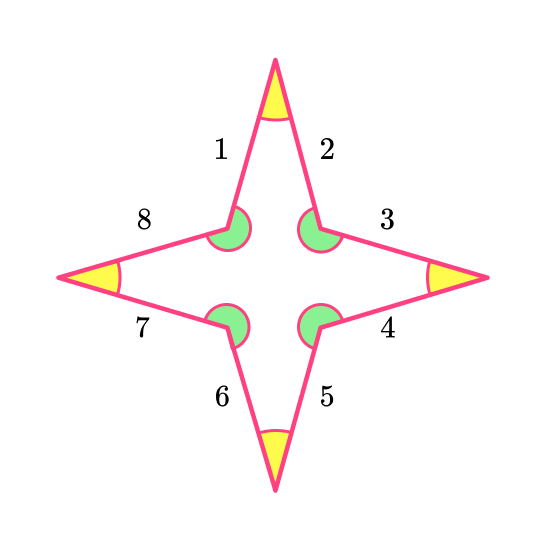An irregular octagon is an 8 sided closed figure that has unequal sides and angles.

## Irregular polygon FAQs

Are the exterior angles of a polygon also congruent?

Just like the interior angles of a polygon, the exterior angles of regular polygons are all congruent and for irregular polygons they are not all congruent.

Can you calculate the area of an irregular polygon?

Yes, you break the shape up into common shapes that have an area formula, such as rectangles and triangles. Then you find the area of each part and add all the parts together to find the total area.

What is the difference between a concave polygon and a convex polygon?

A concave polygon has one or more interior angles that are greater than 180 degrees. A convex polygon has interior angles that are all less than 180 degrees.

What is a regular polygon?

A polygon with equal side lengths and equal angles. An example is an equilateral triangle.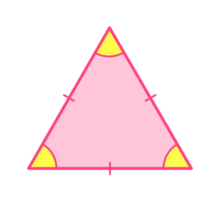## Still stuck?

At Third Space Learning, we specialize in helping teachers and school leaders to provide personalized math support for more of their students through high-quality, online one-on-one math tutoring delivered by subject experts.

Each week, our tutors support thousands of students who are at risk of not meeting their grade-level expectations, and help accelerate their progress and boost their confidence.